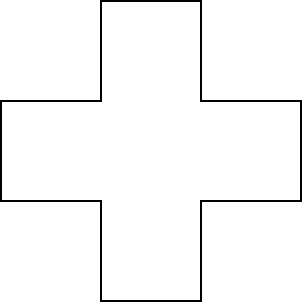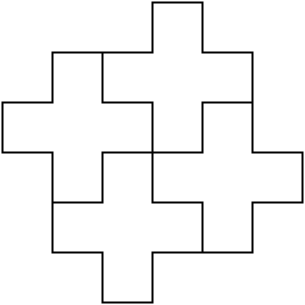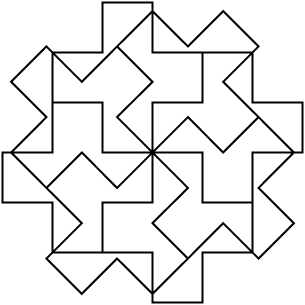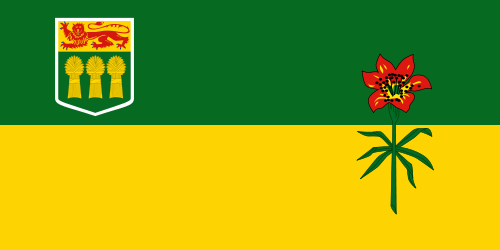# 2. Python Review¶

## 2.2. Converting Between Data Types¶

Construct a block of code that correctly takes in a number from a user, then prints out twice the value that was given.

The following example is not going to work when you try to run it. Try entering in `16`, then `15`. Notice that nothing is printed either time, even though it looks like the conditional should be causing it to print. Can you figure out what is wrong and fix it? Hint: think about data types!

## 2.3. Math Operators¶

If you have trouble with any of the following, you might want to look back at the Math Operators list.

## 2.4. `if`¶

Note

A single equal sign `=` is used to assign a value. Two equal signs `==` are used when comparing a value.

## 2.6. `while` loop¶

Construct a block of code that correctly counts from 10 down to 1, then prints Blastoff!

## 2.7. `for` loop¶

Construct a block of code that correctly counts from 1 up to 10, then prints `Made it!`

Construct a block of code that correctly prints out a greeting for each person, using a for loop.

## 2.9. Functions¶

Construct a function which returns the type of clothing you should wear, based on the parameter temperature. If the temperature is below -10, you will wear a parka and toque. If the temperature is between -10 and 0, wear a toque. If the temperature is greater than 0 but less than 10, wear a sweater. If the temperature is between 10 and 20, wear a t-shirt. If the temperature is greater than 20, wear shorts.

Note

The only thing you need to do for this question is to complete the function definition! You do not need to call the function, as that will be done automatically for you.

The parameter `the_number` needs to be doubled, but only if `the_number` is positive. Return the doubled value of the number that is passed in if `the_number` is positive. If `the_number` is negative, return -1. If `the_number` is 0, return 0.

`double_it_positive(5) → 10`

`double_it_positive(0) → 0`

`double_it_positive(-4) → -1`

## 2.10. Drawing Images Practice¶Construct a function that draws the image shown above:

Draw the following image. Be sure to define and use at least one function as part of your solution.Once you have completed the shape above, try the following. Use the code you made above as a starting point.## 2.11. Image Manipulation with Nested Loops¶Construct a function that draws the negative of the image shown above. Note: due to technical limitations with this question, you need to use the x coordinate as your outer loop.

## 2.12. Strings¶

Construct a block of code that correctly creates a function with a single parameter word that returns the even letters of the word, then calls the function and prints the result.

## 2.13. Lists¶

Note

The only thing you need to do for this question is to complete the function definition! You do not need to call the function, as that will be done automatically for you.

Write a function to count how many odd numbers are in a list.

Examples:

`count_odds([1,3,5,7,9]) → 5`

`count_odds([1,2,3,4,5]) → 3`

`count_odds([2,4,6,8,10]) → 0`

## 2.14. Number Guessing Game¶

Implement a number guessing game in Python that does the following:

• generate a random number from 1 to 100 and stores it in a variable

• repeat the following until the user guesses the number:

• get the user to guess the number

• tell the user if the number is too high or too low

• congratulate the user when they guess the correct number with a message such as “Way to go! You guessed the right number in 9 tries!”

Next Section - Underlying Technology of Computing Devices and the Internet